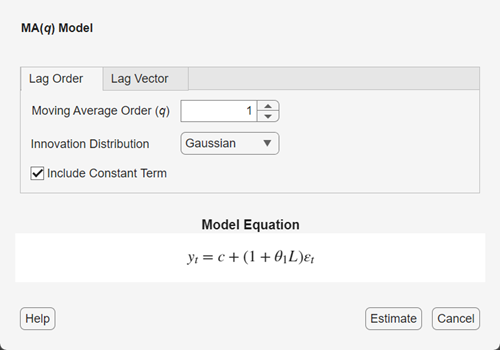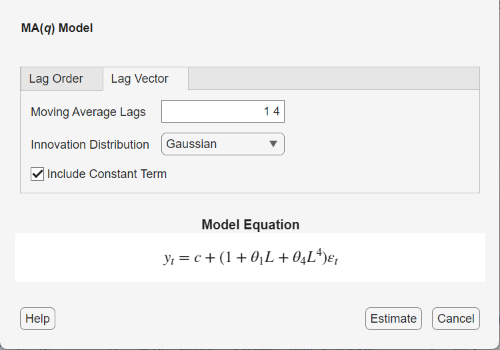MA Model Specifications

Default MA Model

This example shows how to use the shorthand arima(p,D,q) syntax to specify the default MA

${y}_{t}=c+{\epsilon }_{t}+{\theta }_{1}{\epsilon }_{t-1}+\dots +{\theta }_{q}{\epsilon }_{t-q}.$

By default, all parameters in the created model object have unknown values, and the innovation distribution is Gaussian with constant variance.

Specify the default MA(3) model:

Mdl = arima(0,0,3)
Mdl =
arima with properties:

Description: "ARIMA(0,0,3) Model (Gaussian Distribution)"
Distribution: Name = "Gaussian"
P: 0
D: 0
Q: 3
Constant: NaN
AR: {}
SAR: {}
MA: {NaN NaN NaN} at lags [1 2 3]
SMA: {}
Seasonality: 0
Beta: [1×0]
Variance: NaN

The output shows that the created model object, Mdl, has NaN values for all model parameters: the constant term, the MA coefficients, and the variance. You can modify the created model object using dot notation, or input it (along with data) to estimate.

MA Model with No Constant Term

This example shows how to specify an MA(q) model with constant term equal to zero. Use name-value syntax to specify a model that differs from the default model.

Specify an MA(2) model with no constant term,

${y}_{t}={\epsilon }_{t}+{\theta }_{1}{\epsilon }_{t-1}+{\theta }_{2}{\epsilon }_{t-2},$

where the innovation distribution is Gaussian with constant variance.

Mdl = arima('MALags',1:2,'Constant',0)
Mdl =
arima with properties:

Description: "ARIMA(0,0,2) Model (Gaussian Distribution)"
Distribution: Name = "Gaussian"
P: 0
D: 0
Q: 2
Constant: 0
AR: {}
SAR: {}
MA: {NaN NaN} at lags [1 2]
SMA: {}
Seasonality: 0
Beta: [1×0]
Variance: NaN

The MALags name-value argument specifies the lags corresponding to nonzero MA coefficients. The property Constant in the created model object is equal to 0, as specified. The model object has default values for all other properties, including NaN values as placeholders for the unknown parameters: the MA coefficients and scalar variance.

You can modify the created model variable, or input it (along with data) to estimate.

MA Model with Nonconsecutive Lags

This example shows how to specify an MA(q) model with nonzero coefficients at nonconsecutive lags.

Specify an MA(4) model with nonzero MA coefficients at lags 1 and 4 (an no constant term),

${y}_{t}={\epsilon }_{t}+{\theta }_{1}{\epsilon }_{t-1}+{\theta }_{12}{\epsilon }_{t-12},$

where the innovation distribution is Gaussian with constant variance.

Mdl = arima('MALags',[1,4],'Constant',0)
Mdl =
arima with properties:

Description: "ARIMA(0,0,4) Model (Gaussian Distribution)"
Distribution: Name = "Gaussian"
P: 0
D: 0
Q: 4
Constant: 0
AR: {}
SAR: {}
MA: {NaN NaN} at lags [1 4]
SMA: {}
Seasonality: 0
Beta: [1×0]
Variance: NaN

The output shows the nonzero AR coefficients at lags 1 and 4, as specified. The property Q is equal to 4, the number of presample innovations needed to initialize the MA model. The unconstrained parameters are equal to NaN.

Display the value of MA:

Mdl.MA
ans=1×4 cell array
{[NaN]}    {}    {}    {[NaN]}

The MA cell array returns four elements. The first and last elements (corresponding to lags 1 and 4) have value NaN, indicating these coefficients are nonzero and need to be estimated or otherwise specified by the user. arima sets the coefficients at interim lags equal to zero to maintain consistency with MATLAB® cell array indexing.

MA Model with Known Parameter Values

This example shows how to specify an MA(q) model with known parameter values. You can use such a fully specified model as an input to simulate or forecast.

Specify the MA(4) model

${y}_{t}=0.1+{\epsilon }_{t}+0.7{\epsilon }_{t-1}+0.2{\epsilon }_{t-4},$

where the innovation distribution is Gaussian with constant variance 0.15.

Mdl = arima('Constant',0.1,'MA',{0.7,0.2},...
'MALags',[1,4],'Variance',0.15)
Mdl =
arima with properties:

Description: "ARIMA(0,0,4) Model (Gaussian Distribution)"
Distribution: Name = "Gaussian"
P: 0
D: 0
Q: 4
Constant: 0.1
AR: {}
SAR: {}
MA: {0.7 0.2} at lags [1 4]
SMA: {}
Seasonality: 0
Beta: [1×0]
Variance: 0.15

All parameter values are specified, that is, no object property is NaN-valued.

MA Model with t Innovation Distribution

This example shows how to specify an MA(q) model with a Student's t innovation distribution.

Specify an MA(2) model with no constant term,

${y}_{t}={\epsilon }_{t}+{\theta }_{1}{\epsilon }_{t-1}+{\theta }_{2}{\epsilon }_{t-2},$

where the innovation process follows a Student's t distribution with eight degrees of freedom.

tdist = struct('Name','t','DoF',8);
Mdl = arima('Constant',0,'MALags',1:2,'Distribution',tdist)
Mdl =
arima with properties:

Description: "ARIMA(0,0,2) Model (t Distribution)"
Distribution: Name = "t", DoF = 8
P: 0
D: 0
Q: 2
Constant: 0
AR: {}
SAR: {}
MA: {NaN NaN} at lags [1 2]
SMA: {}
Seasonality: 0
Beta: [1×0]
Variance: NaN

The value of Distribution is a struct array with field Name equal to 't' and field DoF equal to 8. When you specify the degrees of freedom, they aren't estimated if you input the model to estimate.

Specify MA Model Using Econometric Modeler App

In the Econometric Modeler app, you can specify the lag structure, presence of a constant, and innovation distribution of an MA(q) model by following these steps. All specified coefficients are unknown but estimable parameters.

1. At the command line, open the Econometric Modeler app.

econometricModeler

Alternatively, open the app from the apps gallery (see Econometric Modeler).

2. In the Time Series pane, select the response time series to which the model will be fit.

3. On the Econometric Modeler tab, in the Models section, click .

The MA Model Parameters dialog box appears.4. Specify the lag structure. To specify an MA(q) model that includes all MA lags from 1 through q, use the Lag Order tab. For the flexibility to specify the inclusion of particular lags, use the Lag Vector tab. For more details, see Specifying Lag Operator Polynomials Interactively. Regardless of the tab you use, you can verify the model form by inspecting the equation in the Model Equation section.

For example:

• To specify an MA(3) model that includes a constant, includes the first lag, and has a Gaussian innovation distribution, set Moving Average Order to 3.

• To specify an MA(2) model that includes the first lag, has a Gaussian distribution, but does not include a constant:

1. Set Moving Average Order to 2.

2. Clear the Include Constant Term check box.

• To specify an MA(4) model containing nonconsecutive lags

${y}_{t}={\epsilon }_{t}+{\theta }_{1}{\epsilon }_{t-1}+{\theta }_{4}{\epsilon }_{t-4},$

where εt is a series of IID Gaussian innovations:

1. Click the Lag Vector tab.

2. Set Moving Average Order to 1 4.

3. Clear the Include Constant Term check box.• To specify an MA(2) model that includes the first lag, includes a constant term, and has t-distributed innovations:

1. Set Moving Average Lags to 2.

2. Click the button, then select t.

The degrees of freedom parameter of the t distribution is an unknown but estimable parameter.

After you specify a model, click to estimate all unknown parameters in the model.